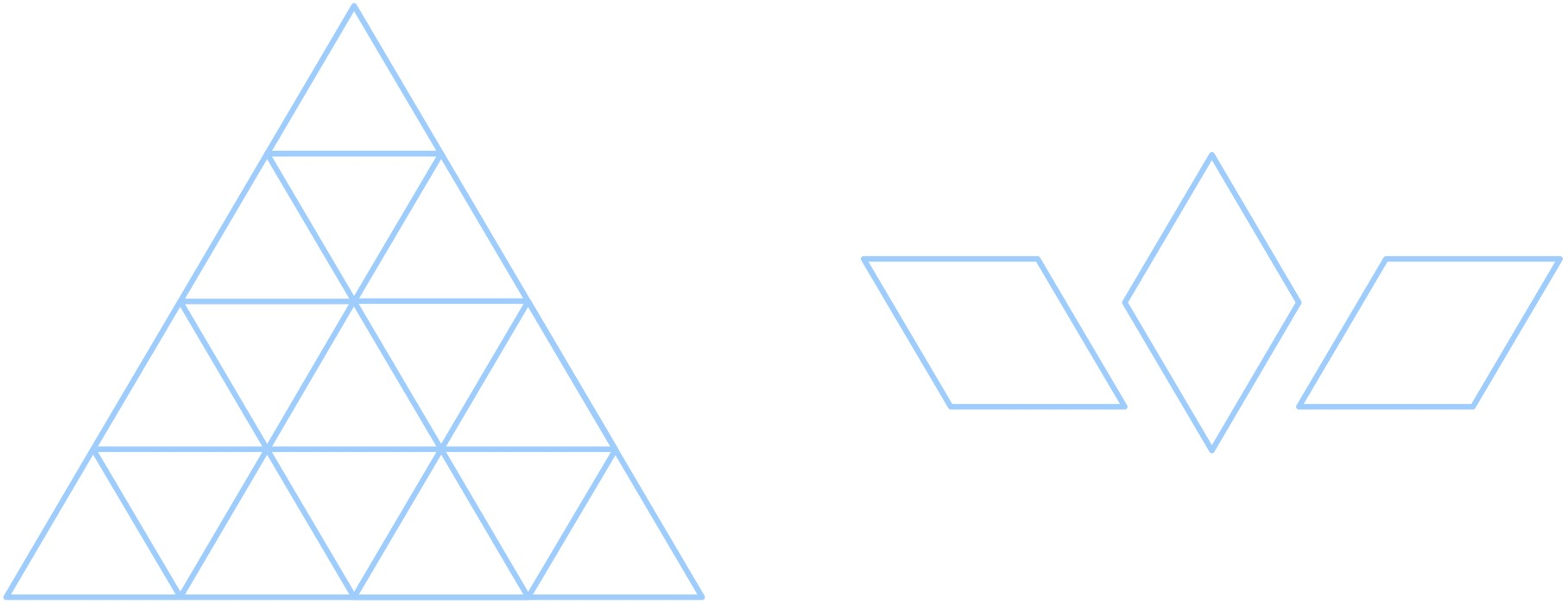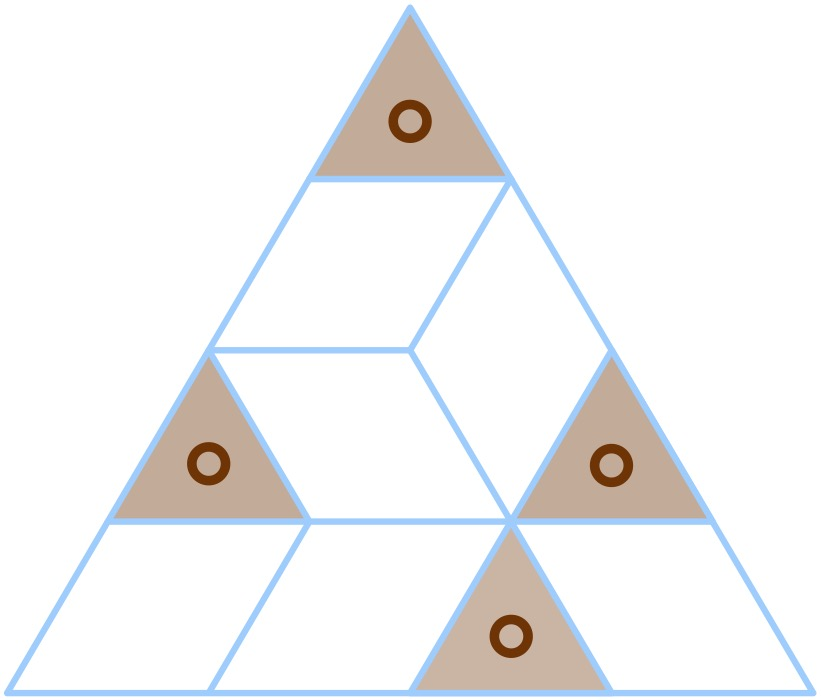시간 제한메모리 제한제출정답맞힌 사람정답 비율
5 초 (추가 시간 없음) 1024 MB4000.000%

## 문제

Chiaki has an equilateral triangle with side length $n$. She would like to tile the triangle using unit rhombi with angles equal to $60^\circ$and $120^\circ$.An equilateral triangle with side length $4$, Three types of rhombus numbered from $1$ to $3$ from left to right.

Chiaki soon figures out it's an impossible task. So she cuts out exactly $n$ of the upward triangles from the equilateral triangle. Now, Chiaki would like to know whether it is possible to tile the remaining shape with rhombi. The figure below is an example tiling for $n=4$.## 입력

There are multiple test cases. The first line of input contains an integer $T$, indicating the number of test cases. For each test case:

The first line contains an integer $n$ ($1 \le n \le 5000$) -- the side length of the equilateral triangle.

The $i$-th line the following $n$ lines contains a binary string $s$ with length $i$, where $s_j=0$ means the $j$-th upward triangle was cut out.

It is guaranteed that the sum of $n$ over all test cases does not exceed $5000$.

## 출력

For each test case,  output a valid tiling. A valid tiling consists of $n$ lines and the $i$-th line contains $i$ characters. The $j$-th character should be:

• '-': if the $j$-th upward triangle was cut out.
• '1': if the $j$-th upward triangle was coverd by the first type rhombus.
• '2': if the $j$-th upward triangle was coverd by the second type rhombus.
• '3': if the $j$-th upward triangle was coverd by the third type rhombus.

If there is no solution, output "Impossible!" (without the quotes) instead.

## 예제 입력 1

1
4
0
11
010
1101


## 예제 출력 1

-
21
-3-
33-1